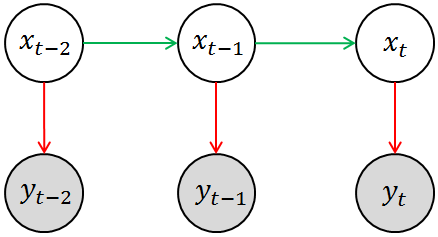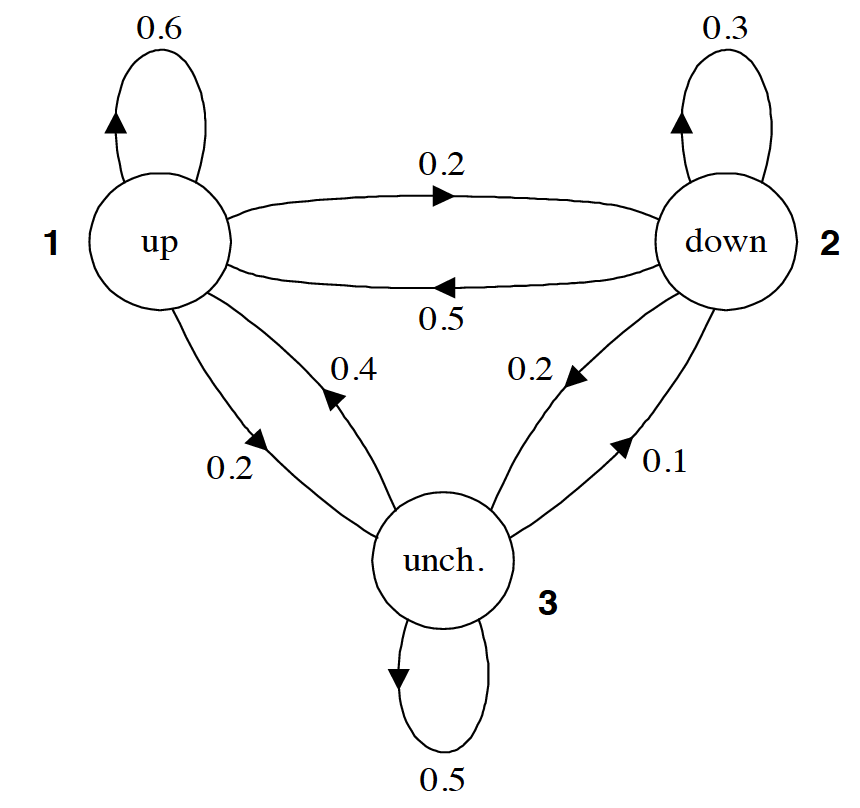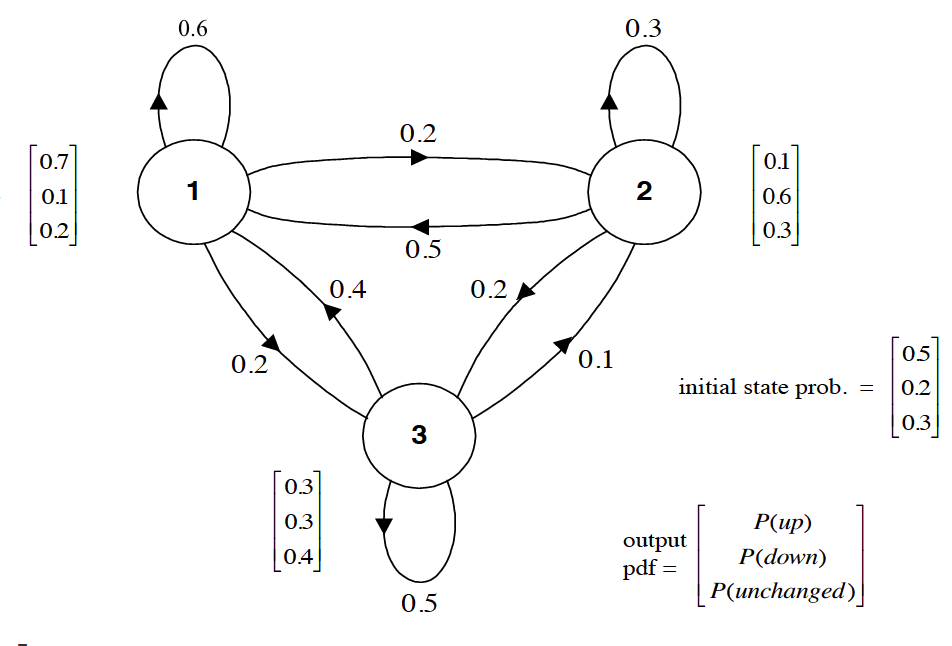# 机器学习中的隐马尔科夫模型（HMM）详解

## 一些必备的数学知识

X(t,w)T×Ω

X(,)$X(\cdot,\cdot)$ 是定义在 T×Ω$T\times \Omega$ 上的二元单值函数。其中 T×Ω$T\times \Omega$ 表示 T$T$Ω$\Omega$ 的笛卡尔积。

P{XtA|Xt1=x1,Xt2=x2,,Xtn=xn}=P{XtA|Xtn=xn}

## 从时间角度考虑不确定性P(xt|xt1,xt2,,x1)=P(xt|xt1)

• 离散的转移概率（Transition Probability）“
P(xt|xt1,xt2,,x1,y1,,yt1)=P(xt|xt1)
• 连续（或离散）的测量概率（Measurement Probability）
P(yt|xt,xt1,,x1,y1,,yt1)=P(yt|xt)

## 一个简单的例子A=0.60.50.40.20.30.10.20.20.5B=0.70.10.30.10.60.30.20.30.4

P(y1,y2,y3)=x1kx2kx3kP(y1,y2,y3,x1,x2,x3)=x1kx2kx3kP(y3|y1,y2,x1,x2,x3)×P(y1,y2,x1,x2,x3)

=x1kx2kx3kP(y3|x3)×P(y1,y2,x1,x2,x3)=x1kx2kx3kP(y3|x3)×P(x3|y1,y2,x1,x2)×P(y1,y2,x1,x2)=x1kx2kx3kP(y3|x3)×P(x3|x2)×P(y1,y2,x1,x2)=x1kx2kx3kP(y3|x3)×P(x3|x2)×P(y2|x2)×P(x2|x1)×P(y1|x1)×P(x1)

## 参考文献

 Stuart Russell and Peter Norvig. Artificial Intelligence: A Modern Approach. 3rd Edition.

 徐伟，赵选民，师义民，秦超英，概率论与数理统计（第2版），西北工业大学出版社

 同时推荐悉尼科大徐亦达博士的机器学习公开课中关于HMM的部分

 关于HMM在NLP中的应用，可以参考Speech and Language Processing. Daniel Jurafsky & James H. Martin, 3rd. Chapter 6

©️2019 CSDN 皮肤主题: 精致技术 设计师: CSDN官方博客